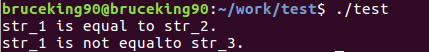• Shell脚本 字符串大小比较 字符串是否相等比较 字符串是否为空判断
分享一下我老师大神的人工智能教程！零基础，通俗易懂！http://blog.csdn.net/jiangjunshow也欢迎大家转载本篇文章。分享知识，造福人民，实现我们中华民族伟大复兴！                字符串比较的方法：相等比较:           $str1 =$str2   //检查str1与str2是否相同           $str1 !=$str2   //检查str1与str2是否不同大小比较:           $str1 <$str2   //检查str1是否小于str2           $str1 >$str2    //检查str1是否大于str2判断字符串是否为空:          -n str1          //检查str1的长度是否大于0          -z str1          //检查str1的长度是否为0注意：：        上面的6个字符串比较。“所有的符号两侧都有空格”，必须小心使用不然将会出现语法错误。同时字符串大小的比较，不能向上面的那么简单，因为shell不知道>是大于还是输出重定向，<是小于还是输入重定向。 所以，<和>必须进行转义。       所以字符串大小的比较使用if [ $val1 \>$val2 ]//中括号两边必须加空格，符号两边必须加空格。            给我老师的人工智能教程打call！http://blog.csdn.net/jiangjunshow展开全文• public static void main(String[] args) { // TODO Auto-generated method stub  //两个字符串 String to="1234567"; String on="123456";  //比较大小 if(to.equals(on)){ System.out
public static void main(String[] args) {// TODO Auto-generated method stub
//两个字符串String to="1234567";
String on="123456";
//比较大小
if(to.equals(on)){//equalsIgnoreCase忽略英文字母的大小写System.out.println("密码正确，请登录");}else{System.out.println("密码不正确，请重新输入");}
}
结果展开全文• 1，输入一串字符, 打印其中每个字符出现的次数...2，输入两串字符串, 无视空格和大小写, 判断其是否相等 3，输入一个字符串，查找出相同字符中 间距最大的那个，并打印出字符和间距，(注: 以遇到的第一个相同字符为准）
文章目录一、记录每个字符出现的次数二、比较字符串是否相等三、找字符串中相同字符的最大间距
一、记录每个字符出现的次数

输入一串字符, 打印其中每个字符出现的次数(如果没有则不显示)

1，实现代码
#include <iostream>
#include <string>
using namespace std;
///储存次数
int arr;
///字符串
string str;

int main()
{
///输入
cout << "样例输入：";
getline(cin, str);
int len = str.length();
///记录次数
//rep(i, 0, len - 1) arr[str[i]] ++;
for (int i = 0; i <= len - 1; i++)
arr[str[i]] ++;			//使用ASCII码作为数组下标
///输出
cout << endl << "结果：" << endl;
for (char i = 0; i < 127; i++)
if (arr[i] != 0)
cout << "'" << i << "': " << arr[i] << "\t";
return 0;
}

2，运行效果二、比较字符串是否相等

输入两串字符串, 无视空格和大小写, 判断其是否相等

1，实现代码
#include <iostream>
#include<string>
using namespace std;

void transform(string* str, int len); //大小写转换,及删除空格

int main()
{

string str1, str2;
int len1, len2;
cout << "请输入测试字符串：";
getline(cin, str1);

cout << "请输入比较字符串：";
getline(cin, str2);

len1 = str1.length();
len2 = str2.length();

transform(&str1, len1);
transform(&str2, len2);

len1 = str1.length();
len2 = str2.length();

cout << endl << "比较结果：";
if (len1 == len2)
{
for (int i = 0; i < len1; i++)
{
if (str1[i]!=str2[i])
{
cout << "两组字符串不相等" << endl;
}
}
cout << "两组字符串相等" << endl;
}
else
{
cout << "两组字符串不相等" << endl;
}
return 0;
}

void transform(string* str, int len)
{
for (auto it = str->begin(); it != str->end(); it++)
{
if ((*it) == ' ')         //删除空格
{
str->erase(it);
}
if ((*it) >= 'A' && (*it) <= 'Z')     //所有大写转小写
{
(*it) = (*it) + 32;
}
}
}

2，运行效果三、找字符串中相同字符的最大间距

输入一个字符串
查找出相同字符中 间距最大的那个
并打印出字符和间距
(注: 以遇到的第一个相同字符为准）

1，实现代码
#include <iostream>
#include <string>
using namespace std;

string str;

int find(int i) {
int index = i + 1;//功能类似于游标
//循环找同字符
while (str[i] != str[index]) {
//没找到的情况
if (index == str.length()) {
return 0;
}
index++;
}
//从 i 下标开始到下一个同字母的间隔数
return index - i - 1;
}

int main() {
//记录最大间隔
int max = 0;
//暂时的最大间隔
int temp_max = 0;
cout << "输入测试字符串：";
getline(cin, str);

//记录最大间隔所对应的字符
char char_max = 0;

for (int i = 0; i < str.length(); i++) {
temp_max = find(i);		//获取暂时的最大字符间隔
//比较最大
if (temp_max >= max) {
max = temp_max;
char_max = str[i];
}
}
cout << endl << "计算结果：";
cout << char_max << ":" << max << endl;
return 0;
}

2，运行效果如有不足之处，还望指正 1。

如果对您有帮助可以点赞、收藏、关注，将会是我最大的动力 ↩︎


展开全文c++
• C语言提供了几个标准库函数...以下是用strcmp()函数比较字符串的一个例子： #include #include int main(void) { char str_1[] = "abc"; char str_2[] = "abc"; char str_3[] = "ABC"; if (strcmp(str_1, str
c语言提供了几个标准库函数，可以比较两个字符串是否相同。以下是用strcmp()函数比较字符串的一个例子：

#include <stdio.h>
#include <string.h>
int main(void)
{
char str_1[] = "abc";
char str_2[] = "abc";
char str_3[] = "ABC";
if (strcmp(str_1, str_2) == 0)
printf("str_1 is equal to str_2. \n");
else
printf("str_1 is not equal to str_2. \n");
if (strcmp(str_1, str_3) == 0)
printf("str_1 is equal to str_3．\n");
else
printf("str_1 is not equal to str_3．\n");
return 0;
}

上例的打印输出如下所示：strcmp()函数有两个参数，即要比较的两个字符串。strcmp()函数对两个字符串进行大小写敏感的(case-sensitiVe)和字典式的(lexicographic)比较，并返回下列值之一：
----------------------------------------------------
返  回  值         意  义
----------------------------------------------------
<0               第一个字符串小于第二个字符串
0               两个字符串相等    ·
>0               第一个字符串大于第二个字符串
----------------------------------------------------
在上例中，当比较str_1(即“abc”)和str_2(即“abc”)时，strcmp()函数的返回值为0。然而，当比较str_1(即"abc")和str_3(即"ABC")时，strcmp()函数返回一个大于0的值，因为按ASCII顺序字符串“ABC”小于“abc”。
strcmp()函数有许多变体，它们的基本功能是相同的，都是比较两个字符串，但其它地方稍有差别。下表列出了C语言提供的与strcmp()函数类似的一些函数：
-----------------------------------------------------------------
函  数  名                   作  用
-----------------------------------------------------------------
strcmp()         对两个字符串进行大小写敏感的比较
strcmpi()        对两个字符串进行大小写不敏感的比较
stricmp()        同strcmpi()
strncmp()        对两个字符串的一部分进行大小写敏感的比较
strnicmp()       对两个字符串的一部分进行大小写不敏感的比较
-----------------------------------------------------------------
在前面的例子中，如果用strcmpi()函数代替strcmp()函数，则程序将认为字符串“ABC”等于“abc”。


展开全文c语言 函数 标准
• equalsIgnoreCase(str):比较字符串内容是否相等，但是会忽略(不区分大小写)大小写 【示例】 public static void main(String[] args) { String str="TOM"; boolean rs = str.equalsIgnoreCase("tom");...java equals
• C语言-比较字符串是否相等,如下图所示： ①：p++,q++ ②：*p<*q
• c ++比较字符串是否相等In this tutorial, we’ll learn methods to compare strings in C++. Consider a scenario wherein you are required to enter your name and password to login to a particular website. ...
• iOS SDK 本身 也提供了 字符串对比的方法： isEqualToString: 用这个字符串方法时， 要注意的事项： if 的后面必须 是一对括号。既然 isEqualToString: 是一个method， method 的使用 都是通过 中括号 来完成的。...
• C++中对于数字类型的数据我们可以直接使用"=="即可比较是否相等，但是对于字符串比较并不适用。我们可以利用以下的两个函数来比较两个字符串是否相等。 1、strcmp 这是用于ANSI标准字符串的函数(如string和char ...c++
• 比较字符串是否相同。 Input 输入两个字符串。 Output 当字符串完全相同时输出0。不完全相同时，比较对应位置不同字符的ASCII值，前者大于后者输出1反之输出-1。 #include<stdio.h> #include<string.h> ...
• 比较两个字符串 BUF1 和 BUF 所含的字符是否相同相同则 AL 返回 0，不同 AL 返回 1 最后的结果为： 第一行为BUF1的输入，第二行为BUF1的输出，第三行为BUF2的输入，第四行为BUF2的输出，第五行为判断的结果。 ...
• int strcmp ( const char *string1, const char *string2 );大小写敏感。int stricmp ( const char *string1, const char *string2 );大小写不敏感。 正确的写法有两种：result = strcmp(&...
• 比较两个字符串是否相等的shell代码，需要的朋友可以参考下
• 网上看了很多，比如==.equals都不行 还是外国网站给力，发现了方法。 ... 用string_a.localeCompare(string_b) 方法去做； 它会返回3个值 0：字符串相等 -1：字符串javascript string
• 在Java中，可以使用“==”来进行判断字符串是否相等。 示例： String s1="Hello"; String s2="Hello"; //输出返回值 System.out.println(s1==s2)； 这时，我们如果运行一下，会发现会输出...
• java 判断字符串是否相等 分类：java 判断字符串相等我们经常习惯性的写上if(str1==str2)，这种写法在java中可能会带来问题。 java中判断字符串是否相等有两种方法： 1、用“==”运算符，该运算符表示指向...
• 从键盘录入两个字符串，从而比较两个字符串的大小(分析：要想判断两个字符串是否一样大，首先要判断出字符串大小是否相等，其次判断字符串中的每个字符是否相同) package com.ghgj.String01; import java.util....
• /** * 比较是否相同，忽略顺序 * @return */ public static boolean isBrother(String s1,String s2){ //长度是否相同 if(s1.length()!=s2.length()){ return false; ...
• ## C语言：比较两个字符串是否相等

万次阅读 多人点赞 2018-09-24 13:02:23
1) 使用strcmp进行比较 下面通过一个例子进行演示： #include &lt;stdio.h&gt; #include &lt;string.h&gt; int main(void) { char* str1 = "abc"; char* str2 = "abc"; ...strcmp...